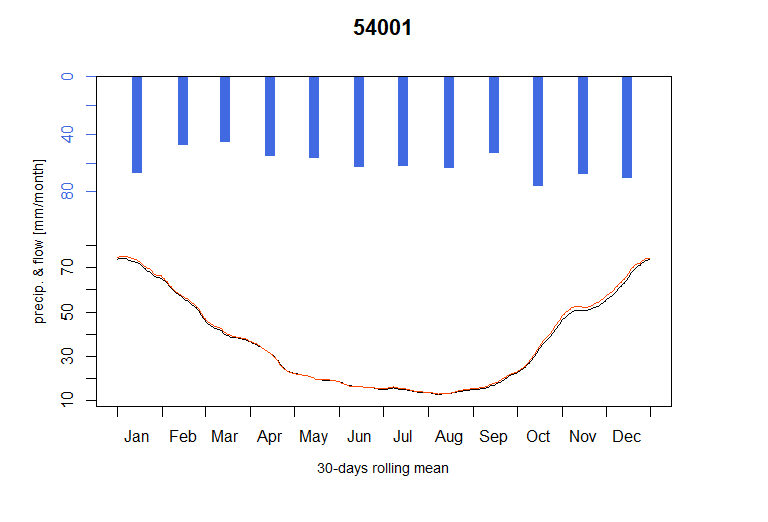# Severn_03: Calibration of an open-loop influenced flow model network

``library(airGRiwrm)``

## Presentation of the study case

The calibration results shown in vignette ‘V02_Calibration_SD_model’ for the flows simulated on the Avon at Evesham (Gauging station ‘54002’) and on the Severn at Buildwas (Gauging station ‘54095’) are not fully satisfactory, especially regarding low flows. These upper basins are actually heavily influenced by impoundments and inter-basin transfers (Higgs and Petts 1988).

To cope with these influences, in this vignette, we will not try to simulate the basin functioning with an hydrological model but we will rather directly inject in the model the observed flows at these nodes.

## Conversion of a gauging station into a release spot

### Modification of the GRiwrm object

The creation of the `GRiwrm` object is detailed in the vignette “V01_Structure_SD_model” of the package. The following code chunk resumes all the necessary steps:

``````data(Severn)
nodes <- Severn\$BasinsInfo[, c("gauge_id", "downstream_id", "distance_downstream", "area")]
nodes\$distance_downstream <- nodes\$distance_downstream
nodes\$model <- "RunModel_GR4J"
griwrm <- CreateGRiwrm(nodes, list(id = "gauge_id", down = "downstream_id", length = "distance_downstream"))``````

To notify the SD model that the provided flows on a node should be directly used instead of an hydrological model, one only needs to declare its model as `NA`:

``````griwrmV03 <- griwrm
griwrmV03\$model[griwrm\$id == "54002"] <- NA
griwrmV03\$model[griwrm\$id == "54095"] <- NA
griwrmV03
#>      id  down length         model    area
#> 1 54057  <NA>     NA RunModel_GR4J 9885.46
#> 2 54032 54057     15 RunModel_GR4J 6864.88
#> 3 54001 54032     45 RunModel_GR4J 4329.90
#> 4 54095 54001     42          <NA> 3722.68
#> 5 54002 54057     43          <NA> 2207.95
#> 6 54029 54032     32 RunModel_GR4J 1483.65``````

Here, we keep the area of this basin which means that the discharge will be provided in mm per time step. If the discharge is provided in m3/s, then the area should be set to `NA` and downstream basin areas should be modified subsequently.

The diagram of the network structure is represented below with:

• in blue, the upstream nodes with a GR4J model
• in green, the intermediate nodes with an SD (GR4J + LAG) model
• in red, the node with direct flow injection (no hydrological model)
``plot(griwrmV03)``

### Generation of the GRiwrmInputsModel object

The formatting of the input data is described in the vignette “V01_Structure_SD_model.” The following code chunk resumes this formatting procedure:

``````BasinsObs <- Severn\$BasinsObs
DatesR <- BasinsObs[]\$DatesR
PrecipTot <- cbind(sapply(BasinsObs, function(x) {x\$precipitation}))
PotEvapTot <- cbind(sapply(BasinsObs, function(x) {x\$peti}))
Precip <- ConvertMeteoSD(griwrm, PrecipTot)
PotEvap <- ConvertMeteoSD(griwrm, PotEvapTot)
Qobs <- cbind(sapply(BasinsObs, function(x) {x\$discharge_spec}))``````

This time, we need to provide observed flows as inputs for the nodes ‘54002’ and ‘54095’:

``QobsInputs <- Qobs[, c("54002", "54095")]``

Then, the `GRiwrmInputsModel` object can be generated taking into account the new `GRiwrm` object:

``````IM_OL <- CreateInputsModel(griwrmV03, DatesR, Precip, PotEvap, QobsInputs)
#> CreateInputsModel.GRiwrm: Treating sub-basin 54001...
#> CreateInputsModel.GRiwrm: Treating sub-basin 54029...
#> CreateInputsModel.GRiwrm: Treating sub-basin 54032...
#> CreateInputsModel.GRiwrm: Treating sub-basin 54057...``````

## Calibration of the new model

Calibration options is detailed in vignette “V02_Calibration_SD_model.” We also apply a parameter regularization here but only where an upstream simulated catchment is available.

The following code chunk resumes this procedure:

``````IndPeriod_Run <- seq(
which(DatesR == (DatesR + 365*24*60*60)), # Set aside warm-up period
length(DatesR) # Until the end of the time series
)
IndPeriod_WarmUp = seq(1,IndPeriod_Run-1)
RunOptions <- CreateRunOptions(IM_OL,
IndPeriod_WarmUp = IndPeriod_WarmUp,
IndPeriod_Run = IndPeriod_Run)
InputsCrit <- CreateInputsCrit(IM_OL,
FUN_CRIT = ErrorCrit_KGE2,
RunOptions = RunOptions, Obs = Qobs[IndPeriod_Run,],
AprioriIds = c("54057" = "54032", "54032" = "54001"),
transfo = "sqrt", k = 0.15
)
CalibOptions <- CreateCalibOptions(IM_OL)``````

The airGR calibration process is applied on each hydrological node of the `GRiwrm` network from upstream nodes to downstream nodes.

``````OC_OL <- suppressWarnings(
Calibration(IM_OL, RunOptions, InputsCrit, CalibOptions))
#> Calibration.GRiwrmInputsModel: Treating sub-basin 54001...
#> Grid-Screening in progress (0% 20% 40% 60% 80% 100%)
#>   Screening completed (243 runs)
#>       Param =   15.000,  247.151,   -2.376,   20.697,    2.384
#>       Crit. KGE2[sqrt(Q)] = 0.9681
#> Steepest-descent local search in progress
#>   Calibration completed (30 iterations, 511 runs)
#>       Param =   19.990,  123.965, -155.531,   10.805,    3.633
#>       Crit. KGE2[sqrt(Q)] = 0.9867
#> Calibration.GRiwrmInputsModel: Treating sub-basin 54029...
#> Grid-Screening in progress (0% 20% 40% 60% 80% 100%)
#>   Screening completed (81 runs)
#>       Param =  247.151,   -0.020,   42.098,    1.944
#>       Crit. KGE2[sqrt(Q)] = 0.9541
#> Steepest-descent local search in progress
#>   Calibration completed (32 iterations, 333 runs)
#>       Param =  214.204,   -0.119,   46.754,    2.022
#>       Crit. KGE2[sqrt(Q)] = 0.9696
#> Calibration.GRiwrmInputsModel: Treating sub-basin 54032...
#> Crit. KGE2[sqrt(Q)] = 0.9867
#>  SubCrit. KGE2[sqrt(Q)] cor(sim, obs, "pearson") = 0.9930
#>  SubCrit. KGE2[sqrt(Q)] cv(sim)/cv(obs)          = 0.9953
#>  SubCrit. KGE2[sqrt(Q)] mean(sim)/mean(obs)      = 1.0102
#>
#> Grid-Screening in progress (0% 20% 40% 60% 80% 100%)
#>   Screening completed (243 runs)
#>       Param =   15.000,  432.681,   -2.376,   83.096,    2.384
#>       Crit. Composite    = 0.9325
#> Steepest-descent local search in progress
#>   Calibration completed (65 iterations, 860 runs)
#>       Param =   19.979,   84.338,   -9.101,  385.564,    3.701
#>       Crit. Composite    = 0.9534
#>  Formula: sum(0.85 * KGE2[sqrt(Q)], 0.15 * GAPX[ParamT])
#> Calibration.GRiwrmInputsModel: Treating sub-basin 54057...
#> Crit. KGE2[sqrt(Q)] = 0.9852
#>  SubCrit. KGE2[sqrt(Q)] cor(sim, obs, "pearson") = 0.9859
#>  SubCrit. KGE2[sqrt(Q)] cv(sim)/cv(obs)          = 1.0009
#>  SubCrit. KGE2[sqrt(Q)] mean(sim)/mean(obs)      = 0.9957
#>
#> Grid-Screening in progress (0% 20% 40% 60% 80% 100%)
#>   Screening completed (243 runs)
#>       Param =   15.000,  169.017,   -2.376,   83.096,    2.384
#>       Crit. Composite    = 0.9368
#> Steepest-descent local search in progress
#>   Calibration completed (38 iterations, 587 runs)
#>       Param =   19.980,   80.601,   -9.592,  279.822,    3.686
#>       Crit. Composite    = 0.9716
#>  Formula: sum(0.85 * KGE2[sqrt(Q)], 0.15 * GAPX[ParamT])
ParamV03 <- sapply(griwrm\$id, function(x) {OC_OL[[x]]\$Param})``````

## Run of the model with this newly calibrated parameters

``````OM_OL <- RunModel(
IM_OL,
RunOptions = RunOptions,
Param = ParamV03
)
#> RunModel.GRiwrmInputsModel: Treating sub-basin 54001...
#> RunModel.GRiwrmInputsModel: Treating sub-basin 54029...
#> RunModel.GRiwrmInputsModel: Treating sub-basin 54032...
#> RunModel.GRiwrmInputsModel: Treating sub-basin 54057...``````

## Plotting of the results

As can be seen below, compared to results of vignette “V02_Calibration_SD_model,” the use of measured flows on upstream influenced basins largely improves the model performance at downstream stations (better low-flow simulations).

``plot(OM_OL, Qobs = Qobs[IndPeriod_Run, ], which = "Regime")``